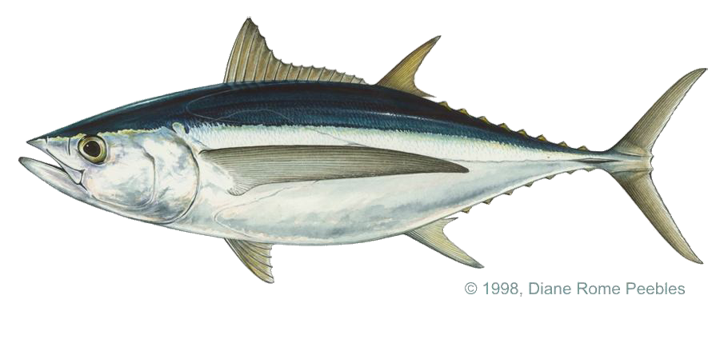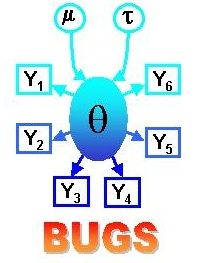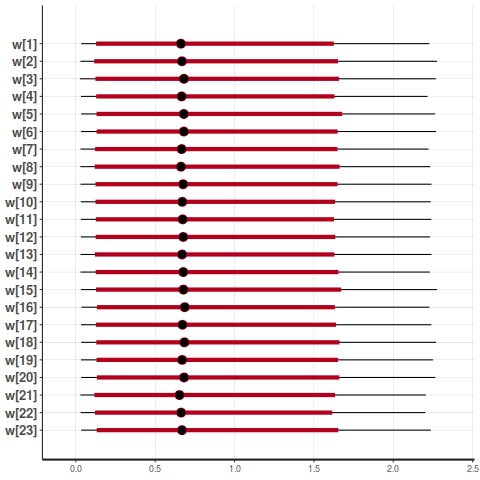## Objective

Translate nonlinear state-space model from BUGS to Stan and diagnose potential problems in the posterior.

Model from two papers:

• Meyer, Renate and Russell B. Millar. 1999. BUGs in Bayesian Stock assessments. Canadian Journal of Fisheries and Aquatic Sciences. 56:1078-1086.
• Millar, Russell B. and Renate Meyer. 2000. Non-linear state space modelling of fisheries biomass dynamics by using Metropolis-Hastings within-Gibbs sampling. Applied Statistics. 49, Part 3: 327-342.

## Model: Data

• $$C_t$$: Catch biomass in year $$t$$
• $$I_t$$: Catch per unit effort in year $$t$$Albacore

## Model: Observation

$$I_t$$ is an index of biomass $$B_t$$. These are related through the parameter $$q$$, catchability.

$I_t = q B_t.$

Nondimensionalizing $$B_t$$ against carrying capacity $$K$$ gives $$P_t = B_t / K$$, observed with log-normal noise with CV $$\tau$$.

\begin{aligned} I_t \mid P_t,q,\tau^2 &= q K P_t e^{v_t}\\ v_t &\sim \operatorname{Normal}(0, \tau^2). \end{aligned}

## Model: Population dynamics

• Schaefer dynamics
• Intrinsic growth rate $$r$$
• log-Normal noise with CV $$\sigma$$
• $$P_1$$ near $$1$$

\begin{aligned} P_1 \mid \sigma^2 &= e^{u_1}\\ P_t \mid P_{t-1},K,r,\sigma^2 &= \left[ P_{t-1} + r P_{t-1}(1 - P_{t-1}) - \frac{C_{t-1}}{K} \right]e^{u_t}\\ u_t &\sim \operatorname{Normal}(0, \sigma^2), \end{aligned}

## Model: Priors

All but $$q$$ at least somewhat informative, based on literature review. Specified $$\log(q) \sim \operatorname{Flat}$$.

\begin{aligned} r &\sim \operatorname{log Normal}(-1.38, 0.51^2)\\ K &\sim \operatorname{log Normal}(5.04, 0.5162^2)\\ p(q) &\propto 1/q\\ \sigma^2 &\sim \operatorname{Inverse Gamma}(3.79, 0.0102)\\ \tau^2 &\sim \operatorname{Inverse Gamma}(1.71, 0.0086). \end{aligned}

## Model: BUGs Approximations

• Truncated priors
• Approximate $$p(q)$$ with inverse-gamma.
• Problems in Stan## Stan: No-U-Turn Sampler

• Hamiltonian dynamics
• Self-tuning

NUTS Simulator

• Divergences: too much curvature
• Max treedepth exceeded: too flat

## Stan: Changes

• Eliminate all truncations
• lognormal takes a standard deviation rather than a precision
• Use Inverse Gamma distribution
• Use specified prior on $$q$$.

## Comparing model parameterizations

• Truncated: Original BUGs model fit in Stan
• Centered
• Noncentered log-Normal process errors
• Noncentered Normal process errors
• Marginalized $$q$$
• Noncentered additive error on catch

## Noncentered log-Normal process errors

\begin{aligned} P_1 \mid \sigma^2 &= u_1 ^ \sigma\\ P_t \mid P_{t-1},\dots &= \bigg[ P_{t-1} + r P_{t-1}(1 - P_{t-1}) - \frac{C_{t-1}}{K} \bigg] u_t ^ \sigma\\ u_t &\sim \operatorname{log-Normal}(0, 1) \end{aligned}

## Noncentered Normal process errors

\begin{aligned} P_1 \mid \sigma^2 &= e^{u_1 \sigma}\\ P_t \mid P_{t-1},\dots &= \bigg[ P_{t-1} + r P_{t-1}(1 - P_{t-1}) - \frac{C_{t-1}}{K} \bigg] \exp(u_t \sigma)\\ u_t &\sim \operatorname{Normal}(0, 1) \end{aligned}

## Marginalized $$q$$

\begin{aligned} Z_i &= \log\left( \frac{I_i}{P_i K} \right)\\ \hat{q}' &= \frac{1}{n} \sum_i Z_i\\ Z_i &\sim \operatorname{Normal}(\hat{q}', \tau^2) \end{aligned}

From Walters & Ludwig (1994), Calculation of Bayes posterior probability distributions for key populations parameters.

\begin{aligned} \nu &\sim \operatorname{Exponential}\left( \frac{\log(0.01)}{2.5} \right)\\ w_t &\sim \operatorname{Normal}(0, \nu^2)\\ C^*_t &= C_t + w_t\\ P_1 \mid \sigma^2 &= e^{u_1}\\ P_t \mid P_{t-1},\dots &= \bigg[ P_{t-1} + r P_{t-1}(1 - P_{t-1}) - \frac{C^*_{t-1}}{K} \bigg] \exp(u_t)\\ u_t &\sim \operatorname{Normal}(0, \sigma^2)\\ \end{aligned}

## Noncentered additive error on catch

\begin{aligned} \nu &\sim \operatorname{Exponential}\left( \frac{\log(0.05)}{2.5} \right)\\ w_t &\sim \operatorname{Normal}(0, 1)\\ C^*_t &= C_t + w_t \nu\\ P_1 \mid \sigma^2 &= e^{u_1}\\ P_t \mid P_{t-1},\dots &= \bigg[ P_{t-1} + r P_{t-1}(1 - P_{t-1}) - \frac{C^*_{t-1}}{K} \bigg] \exp(u_t)\\ u_t &\sim \operatorname{Normal}(0, \sigma^2)\\ \end{aligned}

## Run specifications: full series

• $$4$$ chains
• $$10,000$$ iterations
• $$5,000$$ warmup iterations
• $$20,000$$ inference iterations

## Run specifications: shortened series

• $$4$$ chains
• $$4,000$$ iterations
• $$2,000$$ warmup iterations
• $$8,000$$ inference iterations

## Results: Full series diagnostics

Truncated 0 6394
Centered 0 0 9 0
Noncentered Lognormal 0 52 233 0
Noncentered Normal 0 113 382 0
Marginalized q 0 0 1 0
Explicit catch add 0 0 41 11
Exp catch nc add 0 0 7 0

## Results: shortened series divergences

Truncated 6394 5492 5464 5690
Centered 9 17 8 8
Noncentered Lognormal 233 57 311 1997
Noncentered Normal 382 676 485 1558
Marginalized q 1 18 188 12
Explicit Catch 41 26 12 2
Explicit Catch NC 7 27 9 6

## Results: shortened series divergences

Centered 0 1 0 0
Noncentered Lognormal 0 0 0 0
Noncentered Normal 0 0 1 0
Marginalized q 0 0 0 0
Explicit Catch 11 1 2 0
Explicit Catch NC 0 0 0 0

## Results: timing

Warmup Sampling Min ESS Rate
Truncated 20.3 26.4 13.7
Centered 15.9 19.7 147.0
Noncentered log-Normal 102.9 139.2 17.2
Noncentered Normal 101.2 80.5 32.3
Marginalized q 8.1 8.7 368.6
Explicit Catch 22.5 25.6 10.3
Explicit Catch NC 20.0 22.6 88.6

## Results: timing

Warmup Sampling Min ESS Rate
Centered 29.5 30.1 90.8
Noncentered log-Normal 227.0 197.5 13.8
Noncentered Normal 173.1 163.4 15.2
Marginalized q 15.5 14.6 189.2
Explicit Catch 64.2 89.4 1.8
Explicit Catch NC 37.8 37.3 60.7

## Results: $$w$$## Questions for you

• Other reparameterizations I should try?
• Different priors to use?
• Other ideas?